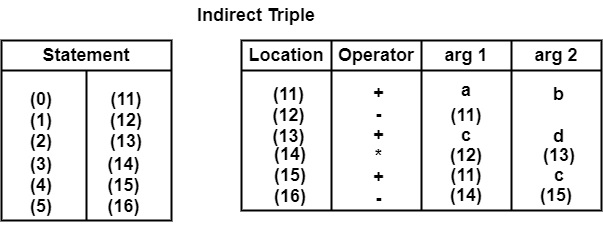# Construct Quadruples, Triples, and Indirect Triples for the expressionn-(a + b) * (c + d) - (a + b + c)

## Solution

First of all this statement will be converted into Three Address Code as−

t1 = a + b

t2 = −t1

t3 = c + d

t4 = t2 ∗ t3

t5 = t1 + c

t6 = t4 − t5

LocationOperatorarg 1arg 2Result
(0)+abt1
(1)t1
t2
(2)+cdt3
(3)t2t3t4
(4)+t1ct5
(5)t4t5t6

Triple

 Location Operator arg 1 arg 2 (0) + a b (1) − (0) (2) + c d (3) ∗ (1) (2) (4) + (0) c (5) − (3) (4)Array Representation

Quadruple is a structure that contains atmost four fields, i.e., operator, Argument 1, Argument 2, and Result. The triples have three fields to represent the three address codes. The field of triples includes the name of the operator, the first source operand, and the second source operand.

This three address code representation contains three (3) fields, i.e., one for operator and two for arguments (i.e., Argument 1 and Argument 2). In this representation, temporary variables are not used. Instead of temporary variables, we use a number in parenthesis to represent a pointer to that particular record of the symbol table.

Quadruples & triples cause some wastage of memory because some fields are not occupied. To prevent wastage of space, the expression can be represented in a single array.

Example− Consider a statement

a = −b + c ∗ d

Its Three Address Code will be

t1 = −b

t2 = c ∗ d

t3 = t1 + t2

a = t3

 Location Operator arg 1 arg 2 Result (0) - b t1 (1) * c d t2 (2) + t1 t2 t3 (3) = t3 A

Since there is wastage of space in the Quadruple, so it can be converted into Array Representation as

 − B t1 ∗ C d t2 + t1 t2 t3 = t3 a